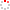#CodeStepByStep## collapse_pairs

Language/Type: PHP arrays traversals

Write a function named `collapse_pairs` that accepts an array of integers as a parameter and returns an array with each of the passed array's pairs of neighboring integers (such as the pair at indexes 0-1, and the pair at indexes 2-3, etc.) combined into a single sum of that pair. The sum will be stored at the even index (0, 2, 4, etc.) if the sum is even and at the odd index (1,3,5, etc.) if the sum is odd. The other index of the pair will change to 0.

For example, if an array named `\$arr` stores the values `[7, 2, 8, 9, 4, 22, 7, 1, 9, 10]`, then the call of `collapse_pairs(\$arr)` should return an array containing the values `[0, 9, 0, 17, 26, 0, 8, 0, 0, 19]`.

The first pair from the original array is collapsed into 9 (7 + 2), which is stored at the odd index 1 because 9 is odd. The second pair is collapsed into 17 (8 + 9), stored at the odd index 3; the third pair is collapsed into 26 (4 + 22), stored at the even index 4; and so on. The figure below summarizes the process for this example array:

```before: index  0   1   2   3   4   5   6   7   8   9
value [7,  2,  8,  9,  4, 22,  7,  1,  9, 10]

\   /   \   /   \   /   \   /   \   /
\ /     \ /     \ /     \ /     \ /
V       V       V       V       V

after:  index  0   1   2   3   4   5   6   7   8   9
value [0,  9,  0, 17, 26,  0,  8,  0,  0, 19]
```

Constraints:

• Do not modify the contents of either array passed to your function
Type your PHP solution code here:

This is a function exercise. Write a PHP function as described. Do not write a complete program; just the function(s) above.